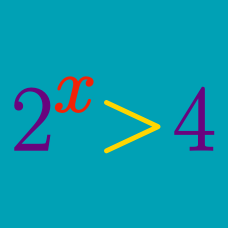Algebra

# Exponential Inequalities - Multiple Terms

Solve the following for $$x:$$ $\left\{\begin{matrix} 3^x > 9 \\ 3^{x^2} \leq 27^{4x}. \end{matrix}\right.$

What is the range of $$x$$ that satisfies the inequality $$\left ( \frac{1}{49} \right )^{x+4} + \left ( \frac{1}{7} \right )^{x+4} > 12 ?$$

What is the range of $$x$$ that satisfies the inequality $$\left(\frac{1}{5}\right)^{3+x} < 625 < \left( \frac{1}{25} \right)^x ?$$

Given $$\log 2=0.3010$$ and $$\log 3=0.4771,$$ solve the inequality $\left(2 ^{7}\right)^x<\left(3 ^x\right)^{6}.$

Given $$n \geq 2,$$ which of the following numbers is the largest: $\sqrt[n]{6^{n-1}}, \sqrt[n]{6^{n+1}}, \sqrt[n+1]{6^n},6 ?$

×